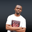Related Tags

euphoria
math.e

# How to check if a number is divisible by 2 in EuphoriaNDUKWE CHIDERA K.

### Overview

Euphoria has some very useful methods built into Euphoria’s standard library packages. They enable us to get simple tasks done in our codes without writing them again.

For example, to check if a particular number is divisible by 2 or not, we can use the is_even() method.

### What is the is_even() method?

To check if a number is divisible by 2 is the same as checking if such a number is an even number. So what the is_even() method does is to check when the integer provided as the argument is divided by 2, will it have a remainder. If it has, then it is not an even number, but if it does not, then it is an even number.

We can even develop our own function to do this hassle-free. But to save developers time and increase productivity, Euphoria has bundled the is_even() method and another helper method as part of the standard library package methods. All we need to do is include the package. We are ready.

### Syntax

is_even(integer_to_check)

• integer_to_check : This integer will be checked to see if it is even or not.

### Return value

The is_even() method returns:

• 1: if the value provided is an even number.

• 0: if the value provided is not an even number.

### Example

In the code below, we’ll check if numbers from 10 to 20 are even or not.

Note: To use this method, we must include the math.e file from standard library std like so include std/math.e

 include std/math.e

for i = 10 to 20 do
? {i, is_even(i)}
end for

### Explanation

Here is a line-by-line explanation of the above code:

• Line 1: We include the math.e file from the standard library files.
• Line 3: The for loop starts and ends at line 5. Each iteration of the loop invokes the is_even() method.
• Line 4: The question mark ? symbol prints the code output on the screen. The is_even() method checks if the numbers provided are even or not.

RELATED TAGS

euphoria
math.e

CONTRIBUTORNDUKWE CHIDERA K.
RELATED COURSES

View all Courses

Keep Exploring

Learn in-demand tech skills in half the time